# Total set

(diff) ← Older revision | Latest revision (diff) | Newer revision → (diff)
A set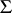of linear functionals on a vector spaceseparating the points of, that is, such that for any non-zero vector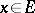there is an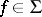with.
More generally, a set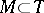, whereis a topological vector space, is called a total set or fundamental set if the linear span ofis dense in. If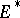, the algebraic dual of, is given the weak topology (so that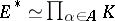, ifis the base field andis an (algebraic) basis for), the two definitions for a set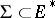agree.